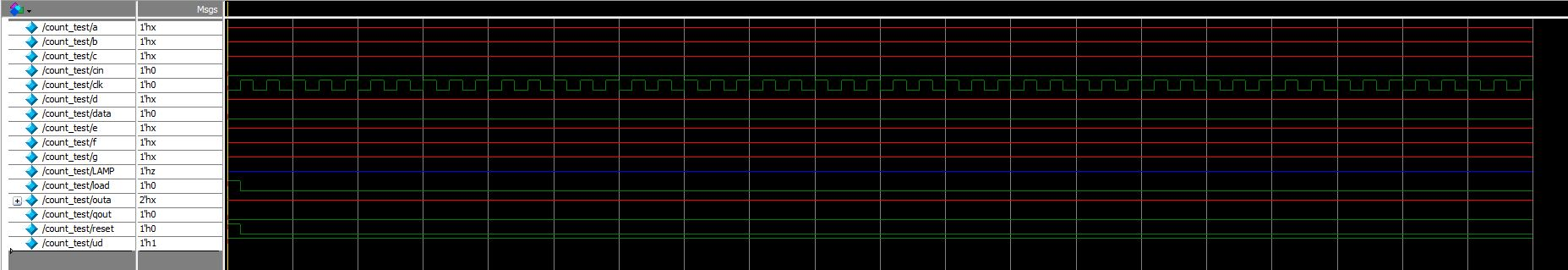verilog简单改错 5C

`````` module count(outa,clk,ud,qout,cout,data,load,cin,reset,a,b,c,d,e,f,g);
output[1:0] outa;
output[19:0] qout;
output cout;
output a,b,c,d,e,f,g;
output LAMP;
input[19:0] data;
wire[1:0] outa;
wire[19:0] qout;
reg reset;
wire LAMP;
reg cin;
reg data;
reg ud;
wire a,b,c,d,e,f,g;

always @(posedge clk)
begin
if(reset==1) outa<=0;
if(cin==0)
begin
if(ud)
outa<=outa+1;
else outa<=outa-1;
end
end
case(outa)
0:begin LAMP=4'b0001;end
1:begin LAMP=4'b0010;end
2:begin LAMP=4'b0100;end
3:begin LAMP=4'b1000;end
default:LAMP=4'b1000;
endcase

always @(posedge clk)
begin
if(reset) qout<=0;
if(cin)
begin
if(ud)
begin
if(outa==0)
begin
if(qout[3:0]==9)
begin
if(qout[19:4]>=1999) qout[19:4]<=1999;
else
begin
qout[3:0]<=0;qout[19:4]<=qout[19:4]+1;
end
end
else
qout[3:0]<=qout[3:0]+1;
end
else if(outa==1)
begin
if(qout[7:4]==9)
begin
if(qout[19:8]>=199) qout[19:8]<=199;
else
begin
qout[7:4]<=0;qout[19:8]<=qout[19:8]+1;
end
end
else
qout[7:4]<=qout[7:4]+1;
end
else if(outa==2)
begin
if(qout[11:8]==9)
begin
if(qout[19:12]>=19) qout[19:12]<=19;
else
begin
qout[11:8]<=0;qout[19:12]<=qout[19:12]+1;
end
end
else
qout[11:8]<=qout[11:8]+1;
end
else
begin
if(qout[15:12]==9)
begin
if(qout[19:16]>=1) qout[19:16]<=1;
else
begin
qout[15:12]<=0;qout[19:16]<=qout[19:16]+1;
end
end
else
qout[15:12]<=qout[15:12]+1;
end
end
else
begin
if(outa==0)
begin
if(qout[3:0]==0)
begin
if([19:4]==0) qout[19:4]<=0;
else
begin
qout[19:4]<=qout[19:4]-1;qout[3:0]<=9;
end
end
else qout[3:0]<=qout[3:0]-1;
end
if(outa==1)
begin
if(qout[7:4]==0)
begin
if([19:8]==0) qout[19:8]<=0;
else
begin
qout[19:8]<=qout[19:8]-1;qout[7:4]<=9;
end
end
else qout[7:4]<=qout[7:4]-1;
end
if(outa==2)
begin
if(qout[11:8]==0)
begin
if([19:12]==0) qout[19:12]<=0;
else
begin
qout[19:12]<=qout[19:12]-1;qout[11:8]<=9;
end
end
else qout[11:8]<=qout[11:8]-1;
end
if(outa==3)
begin
if(qout[15:12]==0)
begin
if([19:16]==0) qout[19:16]<=0;
else
begin
qout[19:16]<=qout[19:16]-1;qout[15:12]<=9;
end
end
else qout[15:12]<=qout[15:12]-1;
end
end
end
end

always @(qout[19:0])
begin
case({qout[19:16],qout[15:12],qout[11:8],qout[7:4],qout[3:0]})
4'd0:{a,b,c,d,e,f,g}=7'b1111110;
4'd1:{a,b,c,d,e,f,g}=7'b0110000;
4'd2:{a,b,c,d,e,f,g}=7'b1101101;
4'd3:{a,b,c,d,e,f,g}=7'b1111001;
4'd4:{a,b,c,d,e,f,g}=7'b0110011;
4'd5:{a,b,c,d,e,f,g}=7'b1011011;
4'd6:{a,b,c,d,e,f,g}=7'b1011111;
4'd7:{a,b,c,d,e,f,g}=7'b1110000;
4'd8:{a,b,c,d,e,f,g}=7'b1111111;
4'd9:{a,b,c,d,e,f,g}=7'b1111011;
default:{a,b,c,d,e,f,g}=7'bx;
endcase
end
endmodule

``````
`````` `timescale 1ns/1ps
module count_test;
reg clk;
wire a,b,c,d,e,f,g;
wire[1:0] outa;
wire qout;
wire LAMP;
initial begin
clk=1'b0;
reset=1'b1;
cin=1'b0;
data=1'b0;
ud=1'b1;
#10
reset=1'b0;

always
#20
clk=~clk;
count(
.clk(clk),
.reset(reset),
.data(data),
.cin(cin),
ud(ud),
);
end
endmodule

``````

-- Compiling module count
** Error: C:/modeltech_10.1a/examples/count.v(12): Port mode is incompatible with declaration: load
** Error: C:/modeltech_10.1a/examples/count.v(14): Port mode is incompatible with declaration: cin
** Error: C:/modeltech_10.1a/examples/count.v(15): Port mode is incompatible with declaration: data
** Error: C:/modeltech_10.1a/examples/count.v(16): Port mode is incompatible with declaration: ud
** Error: C:/modeltech_10.1a/examples/count.v(30): near "=": syntax error, unexpected '=', expecting IDENTIFIER or TYPE_IDENTIFIER
** Error: C:/modeltech_10.1a/examples/count.v(31): near "=": syntax error, unexpected '=', expecting IDENTIFIER or TYPE_IDENTIFIER
** Error: C:/modeltech_10.1a/examples/count.v(32): near "=": syntax error, unexpected '=', expecting IDENTIFIER or TYPE_IDENTIFIER
** Error: C:/modeltech_10.1a/examples/count.v(33): near "=": syntax error, unexpected '=', expecting IDENTIFIER or TYPE_IDENTIFIER
** Error: C:/modeltech_10.1a/examples/count.v(34): near "=": syntax error, unexpected '=', expecting IDENTIFIER or TYPE_IDENTIFIER
** Error: C:/modeltech_10.1a/examples/count.v(104): near "[": syntax error, unexpected '['
** Error: C:/modeltech_10.1a/examples/count.v(116): near "[": syntax error, unexpected '['
** Error: C:/modeltech_10.1a/examples/count.v(128): near "[": syntax error, unexpected '['
** Error: C:/modeltech_10.1a/examples/count.v(140): near "[": syntax error, unexpected '['
** Error: C:/modeltech_10.1a/examples/count.v(146): near "[": syntax error, unexpected '[', expecting IDENTIFIER or TYPE_IDENTIFIER

-- Compiling module count_test
** Error: C:/modeltech_10.1a/examples/test.v(13): near "always": syntax error, unexpected always
** Error: C:/modeltech_10.1a/examples/test.v(17): Named argument not allowed in verilog.

3个回答

(1) module count的port有些沒宣告
(2) always @(posedge clk) begin ...end內應該都是'=>'而非'='
(3) qout[19:16]誤打成[19:16]

1. input 在定义时不可以定义成reg，output可以直接连接到寄存器的输出，但你输入的东西要用register缓存呢？还是要直接连到哪个逻辑上呢？不知道。所以当做wire处理

2. input或者output接口定义完之后，如果要定义它的数据类型（默认是wire），至少宽度要相同。
data是20位，再定义的时候变成1位了
3. always @(posedge clk)这种是给寄存器赋值的写法，作为寄存器是只能阻塞式赋值的，也就是<=，因为寄存器都在时钟的上升沿或者下降沿才生效，不是实时生效的
case语句内的LMAP赋值都改成阻塞式了，包括最后面给abcdefg赋值的方式
4. case语句要放在always语句内部，我猜你28行的end放错位置了
5. 104, 116, 128, 140行的判断条件里来源名称漏了，我猜你要的是qout
6. reset和LAMP作为你的接口，只定义了资源类型，没有定义方向
7. 在always @(posedge clk)语句内操作的只能是reg，也就是寄存器，所以所有跟这些相关的都定义成reg

```````timescale 1ns/1ps
module count_test;
reg clk;
wire a,b,c,d,e,f,g;
wire[1:0] outa;
wire qout;
wire LAMP;
count count(
.outa (outa),
.qout (qout),
.cout (),
.a    (a),
.b    (b),
.c    (c),
.d    (d),
.e    (e),
.f    (f),
.g    (g),
.LAMP (),
.reset(reset),
.clk  (reset),
.ud   (ud),
.cin  (cin),
.data (cin)
);
initial begin
clk=1'b0;
reset=1'b1;
cin=1'b0;
data=1'b0;
ud=1'b1;
#10
reset=1'b0;
end

always
#10
clk=~clk;

endmodule
``````

```````timescale 1ns/1ps
module count_test;
reg clk;
wire a,b,c,d,e,f,g;
wire[1:0] outa;
wire qout;
wire LAMP;
count count(
.outa (outa),
.qout (qout),
.cout (),
.a    (a),
.b    (b),
.c    (c),
.d    (d),
.e    (e),
.f    (f),
.g    (g),
.LAMP (),
.reset(reset),
.clk  (reset),
.ud   (ud),
.cin  (cin),
.data (cin)
);
initial begin
clk=1'b0;
reset=1'b1;
cin=1'b0;
data=1'b0;
ud=1'b1;
#10
reset=1'b0;
end

always
#10
clk=~clk;

endmodule
``````Verilog改错 testbench
-
verilog简单错误修改，求大神，急急急
-
Verilog+niosⅡ 如何实现数字电子钟功能？
-

-
verilog实现I2C接口电路。
-

-
4-16译码器Verilog门级建模该怎么写？
-

-
ISE中verilog的问题（程序较为简单）
-
Verilog VHDL排版插件
-

-

-

-
c++ 读取verilog文件 求助
-
verilog4位加法器仿真
-

-
verilog语言关于memory的问题
-
verilog basys3 计数器设计
-
verilog数码管静态显示计数器
-

2019年9月全国程序员工资统计
2019年9月2日，统计了某招聘网站上的所有程序员招聘信息。并汇总如下。

Spring Cloud（11）——基于RocketMQ的Stream实现

Java 13 新特性全面解读

Java 的每个基本类型都对应了一个包装类型，比如说 int 的包装类型为 Integer，double 的包装类型为 Double。基本类型和包装类型的区别主要有以下 4 点。

500行代码，教你用python写个微信飞机大战

2019诺贝尔经济学奖得主：贫穷的本质是什么？
2019年诺贝尔经济学奖，颁给了来自麻省理工学院的 阿巴希·巴纳吉（Abhijit Vinayak Banerjee）、艾丝特·杜芙若（Esther Duflo）夫妇和哈...
linux：最常见的linux命令（centOS 7.6）